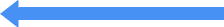# What is a True Score?

#### Psychometricsarnold on Oct 05, 2008

A True Score would be the average of observed scores obtained over an infinite number of repeated testings with the same test.

The essence of Spearman model was in that any observed test score could be envisioned as the composite of two hypothetical components- a true score and a random error component: X=T+E, where X- observed test score, T-individual’s true score and E-random error component.
The classical true score model is:
Observed test score is the true score plus the error score: X = T + E
So, as stated above, the true score is the average of the observed score obtained over an infinite number of repeated testings.
An error score: is the discrepancy between an examinee’s observed test score and his or her true score.
According to classical true score theory, two tests are defined as parallel when:
1. each examinee has the same true score on both forms of the test, and
2. The error variance for the two forms are equal.

Put differently:
According to the classical true score model, an error of measurement is the discrepancy between an examinee’s observed test score (X) and their true score (T). It is a random variable since it is a difference between a random variable, X, and a constant, T. The average of the error scores for an examinee over many repeated testings should be zero.

## DIGITAL MARKETING Email List

##### SPEND ONE HOUR A WEEK ON YOUR DIGITAL MARKETING WITH THE HELP OF OUR WEEKLY GUIDES EMAILED TO YOU!